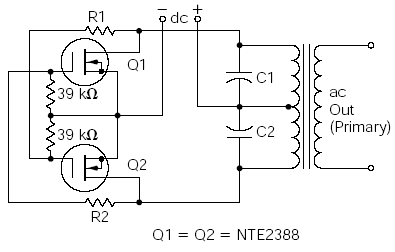Home | Gadget | Fuzzy | Lecturer  | Power  | Transceiver Find more type here... Home Made AC     The circuit illustrated in Fig. 1 is used to convert a low-voltage DC power source (usually 12 volts from an automobile battery) to a higher voltage AC source. Circuits of this type are called inverters. The most common application for this type of circuit is the operation of line powered (120-volt AC) equipment from a car battery. There are, of course, many other applications. For example, if you wanted to use this circuit for the previously mentioned application, the 12-volt DC source from the car battery would be applied to the DC terminals shown in Fig. 1 (observing the correct polarity, and fuse-protecting the 12-volt line from the battery). A standard 12.6-volt ct secondary/120-volt primary power transformer is used. The VA rating of the transformer will depend on the load of the line-powered equipment intended for use with this circuit. If the line-powered device required 120 volts AC at 1 amp (for example), a minimum size of 120 VA is needed (I recommend using at least a 10 to 20% higher VA rating to compensate for certain losses). With the components specified, a 200-VA transformer is the largest transformer that can be used.Figure 1. Power MOSFET Inverter   The combination of C1, C2, and the transformer secondary make up a resonant circuit (resonance will be discussed in a later chapter). Used in conjunction with the active components (Q1 and Q2), this circuit becomes a free-running oscillator, with the frequency of which is determined primarily by the value of C1 and C2. The transformer will operate the most efficiently at about a 60-hertz frequency, so the value of C1 and C2 should be chosen to “tune” the oscillator as closely to that frequency as possible. Capacitors C1 and C2 should be equal in capacitance value. Start with 0.01 uF for C1 and C2, and use a resistance value of 100 K ohms for R1 and R2. These values should bring you close to 60 hertz. If the frequency is too high, decrease the values of the capacitors slightly and vice versa for a lower-frequency condition.